# class TSPlot: public TObject




Overview

A common method used in High Energy Physics to perform measurements is the maximum Likelihood method, exploiting discriminating variables to disentangle signal from background. The crucial point for such an analysis to be reliable is to use an exhaustive list of sources of events combined with an accurate description of all the Probability Density Functions (PDF).

To assess the validity of the fit, a convincing quality check is to explore further the data sample by examining the distributions of control variables. A control variable can be obtained for instance by removing one of the discriminating variables before performing again the maximum Likelihood fit: this removed variable is a control variable. The expected distribution of this control variable, for signal, is to be compared to the one extracted, for signal, from the data sample. In order to be able to do so, one must be able to unfold from the distribution of the whole data sample.

The TSPlot method allows to reconstruct the distributions for the control variable, independently for each of the various sources of events, without making use of any a priori knowledge on this variable. The aim is thus to use the knowledge available for the discriminating variables to infer the behaviour of the individual sources of events with respect to the control variable.

TSPlot is optimal if the control variable is uncorrelated with the discriminating variables.

A detail description of the formalism itself, called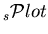, is given in .

The method

Thetechnique is developped in the above context of a maximum Likelihood method making use of discriminating variables.

One considers a data sample in which are merged several species of events. These species represent various signal components and background components which all together account for the data sample. The different terms of the log-Likelihood are:

•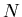: the total number of events in the data sample,
•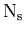: the number of species of events populating the data sample,
•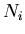: the number of events expected on the average for the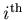species,
•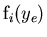: the value of the PDFs of the discriminating variables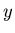for the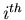species and for event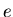,
•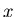: the set of control variables which, by definition, do not appear in the expression of the Likelihood function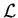.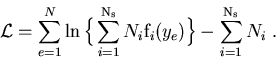(1)

From this expression, after maximization ofwith respect to theparameters, a weight can be computed for every event and each species, in order to obtain later the true distribution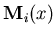of variable. If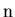is one of thespecies present in the data sample, the weight for this species is defined by: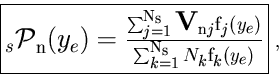(2)

where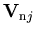is the covariance matrix resulting from the Likelihood maximization. This matrix can be used directly from the fit, but this is numerically less accurate than the direct computation: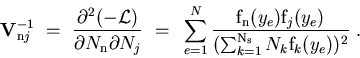(3)

The distribution of the control variableobtained by histogramming the weighted events reproduces, on average, the true distribution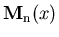.

The class TSPlot allows to reconstruct the true distributionof a control variablefor each of thespecies from the sole knowledge of the PDFs of the discriminating variables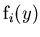. The plots obtained thanks to the TSPlot class are called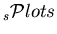.

Some properties and checks

Beside reproducing the true distribution,bear remarkable properties:

• Each-distribution is properly normalized: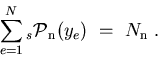(4)

• For any event: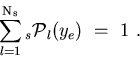(5)

That is to say that, summing up the, one recovers the data sample distribution in, and summing up the number of events entering in afor a given species, one recovers the yield of the species, as provided by the fit. The property 4 is implemented in the TSPlot class as a check.
• the sum of the statistical uncertainties per bin(6)

reproduces the statistical uncertainty on the yield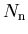, as provided by the fit: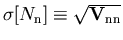. Because of that and since the determination of the yields is optimal when obtained using a Likelihood fit, one can conclude that thetechnique is itself an optimal method to reconstruct distributions of control variables.

Different steps followed by TSPlot

1. A maximum Likelihood fit is performed to obtain the yieldsof the various species. The fit relies on discriminating variablesuncorrelated with a control variable: the later is therefore totally absent from the fit.
2. The weights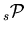are calculated using Eq. (2) where the covariance matrix is taken from Minuit.
3. Histograms ofare filled by weighting the events with.
4. Error bars per bin are given by Eq. (6).
Thereproduce the true distributions of the species in the control variable, within the above defined statistical uncertainties.

Illustrations

To illustrate the technique, one considers an example derived from the analysis wherehave been first used (charmless B decays). One is dealing with a data sample in which two species are present: the first is termed signal and the second background. A maximum Likelihood fit is performed to obtain the two yields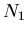and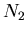. The fit relies on two discriminating variables collectively denotedwhich are chosen within three possible variables denoted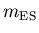,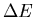and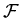. The variable which is not incorporated inis used as the control variable. The six distributions of the three variables are assumed to be the ones depicted in Fig. 1.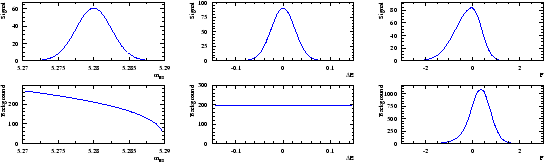A data sample being built through a Monte Carlo simulation based on the distributions shown in Fig. 1, one obtains the three distributions of Fig. 2. Whereas the distribution ofclearly indicates the presence of the signal, the distribution ofandare less obviously populated by signal.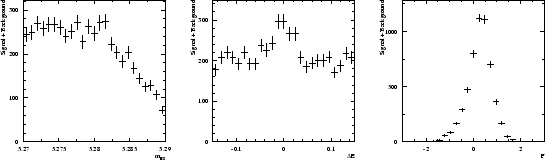Chosingandas discriminating variables to determineandthrough a maximum Likelihood fit, one builds, for the control variablewhich is unknown to the fit, the twofor signal and background shown in Fig. 3. One observes that thefor signal reproduces correctly the PDF even where the latter vanishes, although the error bars remain sizeable. This results from the almost complete cancellation between positive and negative weights: the sum of weights is close to zero while the sum of weights squared is not. The occurence of negative weights occurs through the appearance of the covariance matrix, and its negative components, in the definition of Eq. (2).

A word of caution is in order with respect to the error bars. Whereas their sum in quadrature is identical to the statistical uncertainties of the yields determined by the fit, and if, in addition, they are asymptotically correct, the error bars should be handled with care for low statistics and/or for too fine binning. This is because the error bars do not incorporate two known properties of the PDFs: PDFs are positive definite and can be non-zero in a given x-bin, even if in the particular data sample at hand, no event is observed in this bin. The latter limitation is not specific to, rather it is always present when one is willing to infer the PDF at the origin of an histogram, when, for some bins, the number of entries does not guaranty the applicability of the Gaussian regime. In such situations, a satisfactory practice is to attach allowed ranges to the histogram to indicate the upper and lower limits of the PDF value which are consistent with the actual observation, at a given confidence level.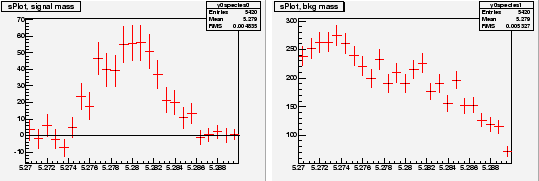Chosingandas discriminating variables to determineandthrough a maximum Likelihood fit, one builds, for the control variablewhich is unknown to the fit, the twofor signal and background shown in Fig. 4. In thefor signal one observes that error bars are the largest in theregions where the background is the largest.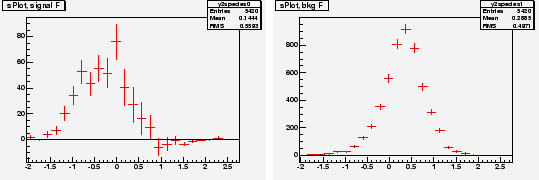The results above can be obtained by running the tutorial TestSPlot.C

## Function Members (Methods)

public:
 TSPlot() TSPlot(Int_t nx, Int_t ny, Int_t ne, Int_t ns, TTree* tree) virtual ~TSPlot() void TObject::AbstractMethod(const char* method) const virtual void TObject::AppendPad(Option_t* option = "") virtual void Browse(TBrowser* b) static TClass* Class() virtual const char* TObject::ClassName() const virtual void TObject::Clear(Option_t* = "") virtual TObject* TObject::Clone(const char* newname = "") const virtual Int_t TObject::Compare(const TObject* obj) const virtual void TObject::Copy(TObject& object) const virtual void TObject::Delete(Option_t* option = "") virtual Int_t TObject::DistancetoPrimitive(Int_t px, Int_t py) virtual void TObject::Draw(Option_t* option = "") virtual void TObject::DrawClass() const virtual TObject* TObject::DrawClone(Option_t* option = "") const virtual void TObject::Dump() const virtual void TObject::Error(const char* method, const char* msgfmt) const virtual void TObject::Execute(const char* method, const char* params, Int_t* error = 0) virtual void TObject::Execute(TMethod* method, TObjArray* params, Int_t* error = 0) virtual void TObject::ExecuteEvent(Int_t event, Int_t px, Int_t py) virtual void TObject::Fatal(const char* method, const char* msgfmt) const void FillSWeightsHists(Int_t nbins = 50) void FillXvarHists(Int_t nbins = 100) void FillYpdfHists(Int_t nbins = 100) void FillYvarHists(Int_t nbins = 100) virtual TObject* TObject::FindObject(const char* name) const virtual TObject* TObject::FindObject(const TObject* obj) const virtual Option_t* TObject::GetDrawOption() const static Long_t TObject::GetDtorOnly() virtual const char* TObject::GetIconName() const virtual const char* TObject::GetName() const Int_t GetNevents() Int_t GetNspecies() virtual char* TObject::GetObjectInfo(Int_t px, Int_t py) const static Bool_t TObject::GetObjectStat() virtual Option_t* TObject::GetOption() const void GetSWeights(TMatrixD& weights) void GetSWeights(Double_t* weights) TH1D* GetSWeightsHist(Int_t ixvar, Int_t ispecies, Int_t iyexcl = -1) TObjArray* GetSWeightsHists() virtual const char* TObject::GetTitle() const TString* GetTreeExpression() TString* GetTreeName() TString* GetTreeSelection() virtual UInt_t TObject::GetUniqueID() const TH1D* GetXvarHist(Int_t ixvar) TObjArray* GetXvarHists() TH1D* GetYpdfHist(Int_t iyvar, Int_t ispecies) TObjArray* GetYpdfHists() TH1D* GetYvarHist(Int_t iyvar) TObjArray* GetYvarHists() virtual Bool_t TObject::HandleTimer(TTimer* timer) virtual ULong_t TObject::Hash() const virtual void TObject::Info(const char* method, const char* msgfmt) const virtual Bool_t TObject::InheritsFrom(const char* classname) const virtual Bool_t TObject::InheritsFrom(const TClass* cl) const virtual void TObject::Inspect() const void TObject::InvertBit(UInt_t f) virtual TClass* IsA() const virtual Bool_t TObject::IsEqual(const TObject* obj) const virtual Bool_t IsFolder() const Bool_t TObject::IsOnHeap() const virtual Bool_t TObject::IsSortable() const Bool_t TObject::IsZombie() const virtual void TObject::ls(Option_t* option = "") const void MakeSPlot(Option_t* option = "v") void TObject::MayNotUse(const char* method) const virtual Bool_t TObject::Notify() static void TObject::operator delete(void* ptr) static void TObject::operator delete(void* ptr, void* vp) static void TObject::operator delete[](void* ptr) static void TObject::operator delete[](void* ptr, void* vp) void* TObject::operator new(size_t sz) void* TObject::operator new(size_t sz, void* vp) void* TObject::operator new[](size_t sz) void* TObject::operator new[](size_t sz, void* vp) TObject& TObject::operator=(const TObject& rhs) virtual void TObject::Paint(Option_t* option = "") virtual void TObject::Pop() virtual void TObject::Print(Option_t* option = "") const virtual Int_t TObject::Read(const char* name) virtual void TObject::RecursiveRemove(TObject* obj) void RefillHist(Int_t type, Int_t var, Int_t nbins, Double_t min, Double_t max, Int_t nspecies = -1) void TObject::ResetBit(UInt_t f) virtual void TObject::SaveAs(const char* filename = "", Option_t* option = "") const virtual void TObject::SavePrimitive(basic_ostream >& out, Option_t* option = "") void TObject::SetBit(UInt_t f) void TObject::SetBit(UInt_t f, Bool_t set) virtual void TObject::SetDrawOption(Option_t* option = "") static void TObject::SetDtorOnly(void* obj) void SetInitialNumbersOfSpecies(Int_t* numbers) void SetNEvents(Int_t ne) void SetNSpecies(Int_t ns) void SetNX(Int_t nx) void SetNY(Int_t ny) static void TObject::SetObjectStat(Bool_t stat) void SetTree(TTree* tree) void SetTreeSelection(const char* varexp = "", const char* selection = "", Long64_t firstentry = 0) virtual void TObject::SetUniqueID(UInt_t uid) virtual void ShowMembers(TMemberInspector& insp, char* parent) virtual void Streamer(TBuffer& b) void StreamerNVirtual(TBuffer& b) virtual void TObject::SysError(const char* method, const char* msgfmt) const Bool_t TObject::TestBit(UInt_t f) const Int_t TObject::TestBits(UInt_t f) const virtual void TObject::UseCurrentStyle() virtual void TObject::Warning(const char* method, const char* msgfmt) const virtual Int_t TObject::Write(const char* name = 0, Int_t option = 0, Int_t bufsize = 0) virtual Int_t TObject::Write(const char* name = 0, Int_t option = 0, Int_t bufsize = 0) const
protected:
 virtual void TObject::DoError(int level, const char* location, const char* fmt, va_list va) const void TObject::MakeZombie() void SPlots(Double_t* covmat, Int_t i_excl)

## Data Members

public:
 enum TObject::EStatusBits { kCanDelete kMustCleanup kObjInCanvas kIsReferenced kHasUUID kCannotPick kNoContextMenu kInvalidObject }; enum TObject::[unnamed] { kIsOnHeap kNotDeleted kZombie kBitMask kSingleKey kOverwrite kWriteDelete };
protected:
 TMatrixD fMinmax mins and maxs of variables for histogramming Int_t fNSpecies Number of species Int_t fNevents Total number of events Double_t* fNumbersOfEvents [fNSpecies] estimates of numbers of events in each species Int_t fNx Number of control variables Int_t fNy Number of discriminating variables TMatrixD fPdfTot ! TMatrixD fSWeights computed sWeights TObjArray fSWeightsHists histograms of weighted variables TString* fSelection Selection on the tree TTree* fTree ! TString* fTreename The name of the data tree TString* fVarexp Variables used for splot TMatrixD fXvar ! TObjArray fXvarHists histograms of control variables TMatrixD fYpdf ! TObjArray fYpdfHists histograms of pdfs TMatrixD fYvar ! TObjArray fYvarHists histograms of discriminating variables

## Class Charts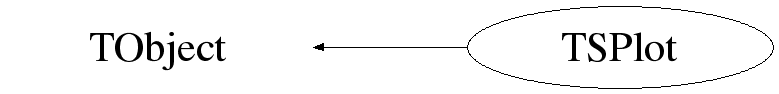## Function documentation

TSPlot()
 default constructor (used by I/O only)

TSPlot(Int_t nx, Int_t ny, Int_t ne, Int_t ns, TTree* tree)
normal TSPlot constructor
nx :  number of control variables
ny :  number of discriminating variables
ne :  total number of events
ns :  number of species
tree: input data

~TSPlot()
 destructor

void Browse(TBrowser* b)
To browse the histograms

void SetInitialNumbersOfSpecies(Int_t* numbers)
Set the initial number of events of each species - used
as initial estimates in minuit

void MakeSPlot(Option_t* option = "v")
Calculates the sWeights
The option controls the print level
"Q" - no print out
"V" - prints the estimated #of events in species - default
"VV" - as "V" + the minuit printing + sums of weights for control

void SPlots(Double_t* covmat, Int_t i_excl)
Computes the sWeights from the covariance matrix

void GetSWeights(TMatrixD &weights)
Returns the matrix of sweights

void GetSWeights(Double_t *weights)
Returns the matrix of sweights. It is assumed that the array passed in the argurment is big enough

void FillXvarHists(Int_t nbins = 100)
Fills the histograms of x variables (not weighted) with nbins


Returns the array of histograms of x variables (not weighted)
been filled, they are filled with default binning 100.

TH1D * GetXvarHist(Int_t ixvar)
Returns the histogram of variable #ixvar
been filled, they are filled with default binning 100.

void FillYvarHists(Int_t nbins = 100)
Fill the histograms of y variables


Returns the array of histograms of y variables. If histograms have not already
been filled, they are filled with default binning 100.

TH1D * GetYvarHist(Int_t iyvar)
Returns the histogram of variable iyvar.If histograms have not already
been filled, they are filled with default binning 100.

void FillYpdfHists(Int_t nbins = 100)
Fills the histograms of pdf-s of y variables with binning nbins


Returns the array of histograms of pdf's of y variables with binning nbins
been filled, they are filled with default binning 100.

TH1D * GetYpdfHist(Int_t iyvar, Int_t ispecies)
Returns the histogram of the pdf of variable #iyvar for species #ispecies, binning nbins
been filled, they are filled with default binning 100.

void FillSWeightsHists(Int_t nbins = 50)
The order of histograms in the array:
x0_species0, x0_species1,..., x1_species0, x1_species1,..., y0_species0, y0_species1,...
If the histograms have already been filled with a different binning, they are refilled
and all histograms are deleted


Returns an array of all histograms of variables, weighted with sWeights
If histograms have not been already filled, they are filled with default binning 50
The order of histograms in the array:
x0_species0, x0_species1,..., x1_species0, x1_species1,..., y0_species0, y0_species1,...

void RefillHist(Int_t type, Int_t var, Int_t nbins, Double_t min, Double_t max, Int_t nspecies = -1)
The Fill...Hist() methods fill the histograms with the real limits on the variables
This method allows to refill the specified histogram with user-set boundaries min and max
Parameters:
type = 1 - histogram of x variable #nvar
= 2 - histogram of y variable #nvar
= 3 - histogram of y_pdf for y #nvar and species #nspecies
= 4 - histogram of x variable #nvar, species #nspecies, WITH sWeights
= 5 - histogram of y variable #nvar, species #nspecies, WITH sWeights

TH1D * GetSWeightsHist(Int_t ixvar, Int_t ispecies, Int_t iyexcl = -1)
Returns the histogram of a variable, weithed with sWeights
If histograms have not been already filled, they are filled with default binning 50
If parameter ixvar!=-1, the histogram of x-variable #ixvar is returned for species ispecies
If parameter ixvar==-1, the histogram of y-variable #iyexcl is returned for species ispecies
If the histogram has already been filled and the binning is different from the parameter nbins
all histograms with old binning will be deleted and refilled.

void SetTree(TTree* tree)
 Set the input Tree

void SetTreeSelection(const char* varexp = "", const char* selection = "", Long64_t firstentry = 0)
Specifies the variables from the tree to be used for splot

Variables fNx, fNy, fNSpecies and fNEvents should already be set!

In the 1st parameter it is assumed that first fNx variables are x(control variables),
then fNy y variables (discriminating variables),
then fNy*fNSpecies ypdf variables (probability distribution functions of dicriminating
variables for different species). The order of pdfs should be: species0_y0, species0_y1,...
species1_y0, species1_y1,...species[fNSpecies-1]_y0...
The 2nd parameter allows to make a cut
TTree::Draw method description contains more details on specifying expression and selection


{ return kTRUE;}

{return fNevents;}

{return fNSpecies;}

{return fTreename;}

{return fSelection;}

{return fVarexp;}
void SetNX(Int_t nx)
{fNx=nx;}
void SetNY(Int_t ny)
{fNy=ny;}
void SetNSpecies(Int_t ns)
{fNSpecies=ns;}
void SetNEvents(Int_t ne)
{fNevents=ne;}

Author: Muriel Pivk, Anna Kreshuk 10/2005
Last update: root/splot:$Id: TSPlot.h 20882 2007-11-19 11:31:26Z rdm$
Copyright (c) 2005 ROOT Foundation, CERN/PH-SFT *

This page has been automatically generated. If you have any comments or suggestions about the page layout send a mail to ROOT support, or contact the developers with any questions or problems regarding ROOT.